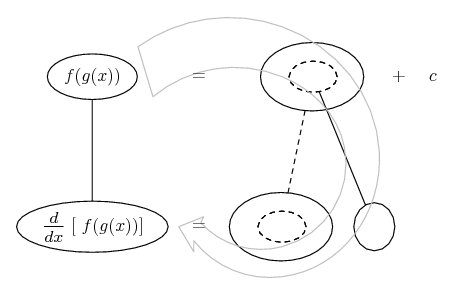# Thread: Derivative of 14x/(1+12.6e^(-0.73x)

1. ## Derivative of 14x/(1+12.6e^(-0.73x)

$\displaystyle f(x)=\frac{14x}{(1+12.6e^{-0.73x})}$

Hello, I'm having some brain freeze on finding the derivative for this problem.

First, I changed the denominator to $\displaystyle {(1+12.6e^{-0.73x})^{-1}$, and then used the Product Rule. $\displaystyle f(x)=\frac{d}{dx}{[14x]}*{(1+12.6e^{-0.73x})^{-1}+\frac{d}{dx}{[(1+12.6e^{-0.73x})^{-1}]*{14x}$

That left me with: $\displaystyle f(x)={}*{(1+12.6e^{-0.73x})^{-1}+{[(12.6e^{-0.73x})^{-1}]*{14x}$

This is where I'm getting brain frozen. I checked the back off the book it says the answer is: $\displaystyle f(x)={}*{(1+12.6e^{-0.73x})^{-1}+ {128.772x(1+12.6e^{-0.73x})^{-2}e^{-0.73x}$.

I'm not sure where the value of $\displaystyle {128.772x(1+12.6e^{-0.73x})^{-2}e^{-0.73x}$ came from. I see parts of the Chain Rule, but the 128.772 has me dumbfounded. If there's a coefficient in front of $\displaystyle {e}$ what happens when taking the derivative of $\displaystyle {e}^{x}$?

2. ## Re: Derivative of 14x/(1+12.6e^(-0.73x)

Sorry about my handwritingThe last step I just used the distributive property

3. ## Re: Derivative of 14x/(1+12.6e^(-0.73x)

During your step here:

$\displaystyle f(x)={}*{(1+12.6e^{-0.73x})^{-1}+{[(12.6e^{-0.73x})^{-1}]*{14x}$

You should show how you used the Chain Rule to see where you made the error, since you never finished the Chain rule on the second term's $\displaystyle [(12.6e^{-0.73x})^{-1}]$

4. ## Re: Derivative of 14x/(1+12.6e^(-0.73x)

If you feel insistent about the Product rule:I thought this might not be clear enough and I was going to make some changes but it's late and I have to sleep so bye for now

5. ## Re: Derivative of 14x/(1+12.6e^(-0.73x)

Hello, AZach!

$\displaystyle f(x)\:=\:\frac{14x}{(1+12.6e^{-0.73x})}$

$\displaystyle \text{Hello, I'm having some brain freeze on finding the derivative for this problem.}$

$\displaystyle \text{First, I changed the denominator to }(1+12.6e^{-0.73x})^{-1},\,\text{ and then used the Product Rule.}$

$\displaystyle f'(x)\:=\:\frac{d}{dx}(14x)\cdot(1+12.6e^{-0.73x})^{-1}+\frac{d}{dx}[(1+12.6e^{-0.73x})^{-1}]\cdot(14x)$

$\displaystyle \text{That left me with: }\:f'(x)\:=\:(14)\cdot{(1+12.6e^{-0.73x})^{-1}+\underbrace{[(12.6e^{-0.73x})^{-1}]}_{\text{This is wrong!}}\cdot(14x)$

The derivative of: .$\displaystyle g(x) \;=\;\left(1+12.6\:\!e^{-0.73x}\right)^{-1}$

. . . . . . . . . . .is: .$\displaystyle g'(x) \;=\;-\left(1 + 12.6\:\!e^{-0.73x}\right)^{-2}\cdot 12.6\:\!e^{-0.73x}\cdot (-0.73)$

. . . . . . . . . . . . . . . . . . $\displaystyle =\;9.198\:\!e^{-0.73x}\left(1 + 12.6\:\!e^{-0.73x}\right)^{-2}$

. . . . . . . . . . . . . . . . . . $\displaystyle =\;\frac{9.198}{e^{0.73x}\left(1 + 12.6\:\!e^{-0.73x}\right)^2}$

Unless you are very good at handling negative exponents,
. . I do not recommend switching to the Product Rule.

6. ## Re: Derivative of 14x/(1+12.6e^(-0.73x)Originally Posted by Soroban. . . . . . . . . . .is: .$\displaystyle g'(x) \;=\;-\left(1 + 12.6\:\!e^{-0.73x}\right)^{-2}\cdot 12.6\:\!e^{-0.73x}\cdot (-0.73)$
First off, thanks for the thorough responses, Daigo and Soroban. For this quoted part, I think this is where one of my main problems has been. I knew the derivative of $\displaystyle {e}^{x}$ was itself, so I ignorantly assumed that a coefficient in front of the 'x' variable wouldn't alter that. Looking at this from a function point of view, is $\displaystyle 12.6e^{-0.73x}$ and $\displaystyle {-0.73x}$ both considered inside functions of $\displaystyle (1+12.6e^{-0.73x})^{-1}$?

7. ## Re: Derivative of 14x/(1+12.6e^(-0.73x)Originally Posted by AZachLooking at this from a function point of view, is $\displaystyle 12.6e^{-0.73x}$ and $\displaystyle {-0.73x}$ both considered inside functions of $\displaystyle (1+12.6e^{-0.73x})^{-1}$?
Absolutely - it's a glass-onion / russian doll situation!

Just in case a picture helps...... where (key in spoiler) ...

Spoiler:... is the chain rule. Straight continuous lines differentiate downwards (integrate up) with respect to the main variable (in this case x), and the straight dashed line similarly but with respect to the dashed balloon expression (the inner function of the composite which is subject to the chain rule).

The general drift is...__________________________________________________ ________

Don't integrate - balloontegrate!

Balloon Calculus; standard integrals, derivatives and methods

Balloon Calculus Drawing with LaTeX and Asymptote!

#### Search Tags

126e073x, 14x or 1, derivative